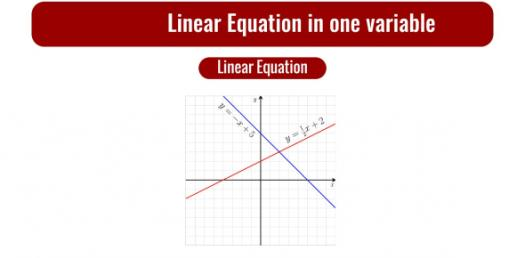# Can You Solve The Basic Linear Equation Quiz?

5 Questions | Attempts: 433SettingsThis quiz revolves around algebra and the linear equation. Algebra, for most people, is a challenging concept to master. You have to know your stuff to be able to pass. Did you know that algebra is the part of mathematics in which letters and other general symbols are used to represent numbers and quantities in the formula and equations? Take this quiz and test your algebra IQ!

• 1.
Which equation describes the relationship between x and y in the table above?
• A.

Y = y + 5

• B.

Y = 2x - 5

• C.

Y = 5x - 2

• D.

Y = x + 2

• 2.
The width and length, in feet, of a rectangular playground are x and x + 2. A fence costs \$17 per foot.  Write an expression to show the distance around the playground.
• A.

X ( x + 2)

• B.

X +( x + 2 )+ x +( x + 2)

• C.

X - x (2)

• D.

X + 17 (x + 2)

• 3.
The width and length, in feet, of a rectangular playground are x and x + 2. A fence costs \$17 per foot.  What is the expression for the cost of the fence?
• A.

17[x + (x + 2)]

• B.

17x(x + 2)

• C.

17[x + (x + 2) + x + (x + 2)]

• D.

17 + x + (x + 2)

• 4.
The width and length, in feet, of a rectangular playground are x and x + 2. A fence costs \$17 per foot and the total cost for the fence was \$1,428.  What  is the value of x?
• A.

20

• B.

84

• C.

2

• D.

8

• 5.
A fence costs \$17 per foot and the total cost for the fence was \$1,428.  How many feet of fence were purchased?
• A.

20

• B.

84

• C.

8

• D.

17

## Related TopicsBack to top
×

Wait!
Here's an interesting quiz for you.